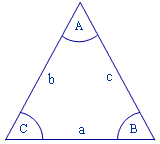up
?

Solve Triangle

Use the following form to solve any given triangle. Three inputs are required for the solution. Atleast one of the input needs to be a side of the triangle.

Input Data

 ab c
Angle A  :  60 degrees
Angle B  :  60 degrees
Angle C  :  60 degrees

How did we get there?

Given:      Sides a = 10, b = 10,  c = 10

Applying Cosine law on side 'a' we get;

CosA = (b2 + c2 – a2 )/2 · b · c

CosA = 0.5, Or A = 60 degrees

Similarly

CosB = (a2 + c2 – b2 )/2 · a · c

CosB = 0.5, Or B = 60 degrees

Finally

CosC = (a2 + b2 – c2 )/2 · a · b

CosC = 0.5, Or C = 60 degrees

Math based tools

Other math based tools